# How to Perform Date Calculations in Windows 10 CalculatorWindows 10 provides us with a very efficient and useful Calculator app, which not only performs the regular arithmetic calculations but it can be used for many other purposes. For example, the Calculator app can also be used to perform all the kinds of date calculations. In this article, we will explain to you the methods through which you can perform the date calculations using the Calculator app in Windows 10.

## Performing the Date Calculations in Windows 10 Calculator

In order to perform the date calculations using the Calculator app in Windows 10, you can make use of any of the following two methods:

### Calculate the difference between two dates using Windows Calculator

In this method, we will tell you how you can calculate the difference between two dates using the Calculator app in Windows 10. For doing this, you will need to perform the following steps:

Type calculator in the search section of your taskbar and click on the search result in order to launch the calculator app. The newly opened Calculator app is shown in the following image: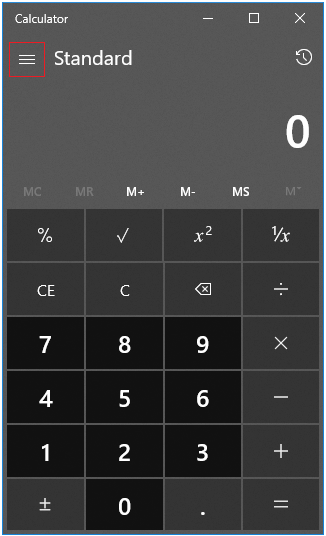Click on the hamburger button as highlighted in the image shown above in order to launch a menu.

Now click on Date Calculation option from this menu as shown in the image below:As soon as you will click on it, you will be able to see a new interface where you will be able to calculate the difference between two dates as shown in the following image: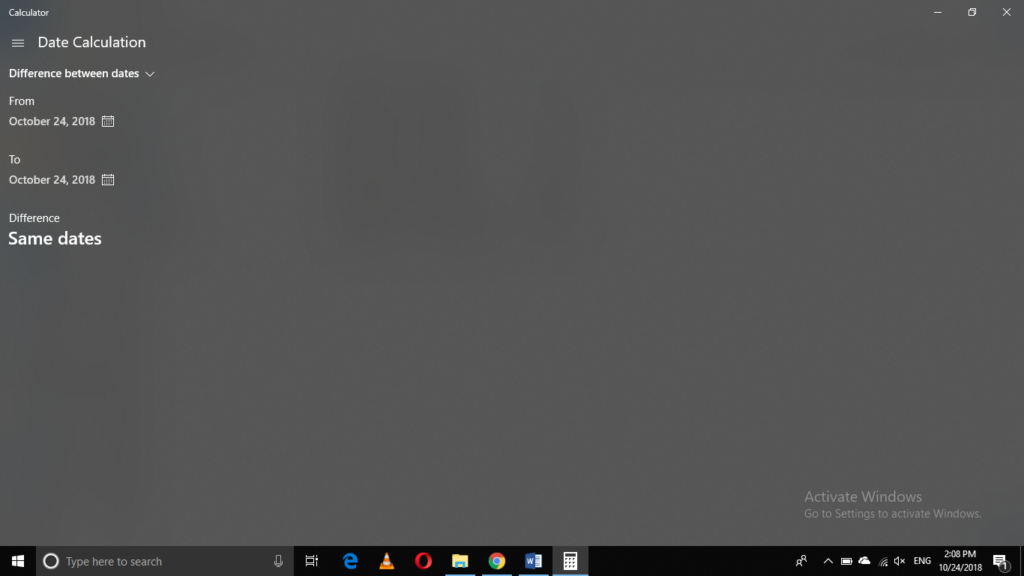You can easily select the two dates whose difference you want to calculate simply by using the calendars associated with the “From” and the “To” fields as shown in the image below: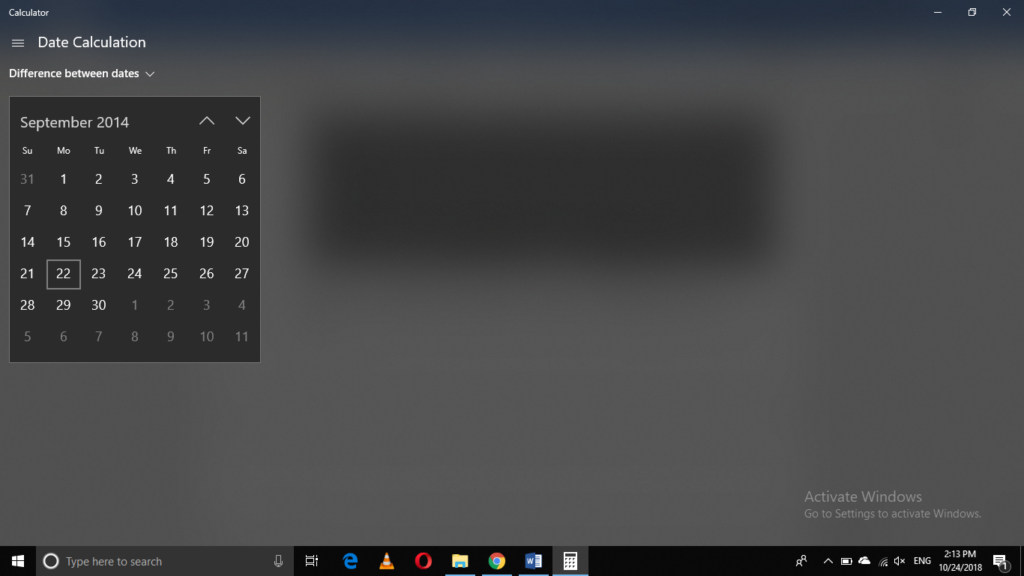As soon as you will select the two dates, the Calculator application will immediately display the difference between these two dates as highlighted in the following image: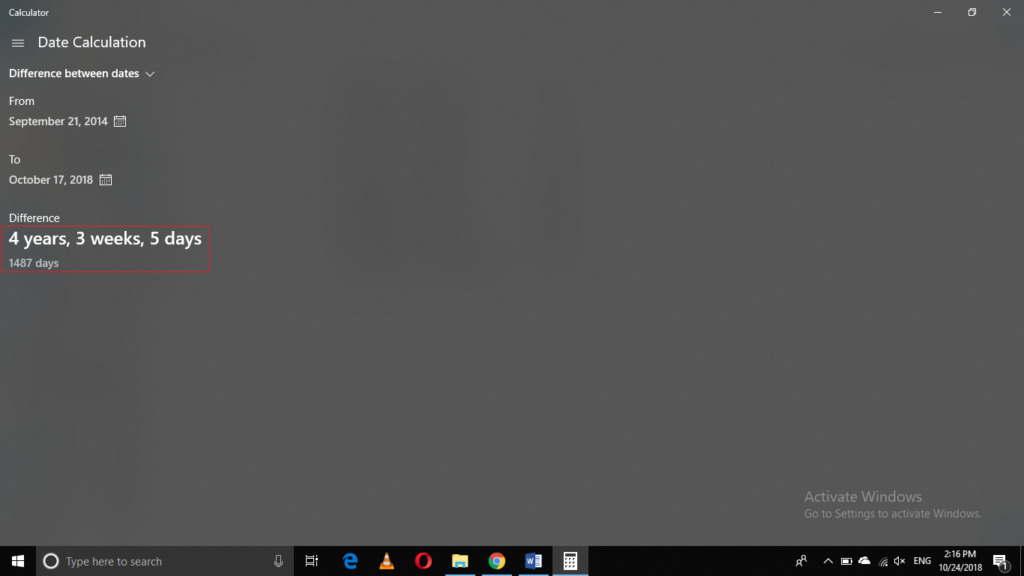### Subtract days to or from a specified date using Windows Calculator

In this method, we will tell you how you can add or subtract days to or from a specified date using the Calculator app in Windows 10. For doing this, you will need to perform the following steps:

Type calculator in the search section of your taskbar and click on the search result in order to launch the calculator app. The newly opened Calculator app is shown in the following image:Click on the hamburger button as highlighted in the image shown above in order to launch a menu.

Now click on Date Calculation option from this menu as shown in the image below:Click on the dropdown list below the field of Date Calculation and select Add or Subtract dates from this list as highlighted in the following image: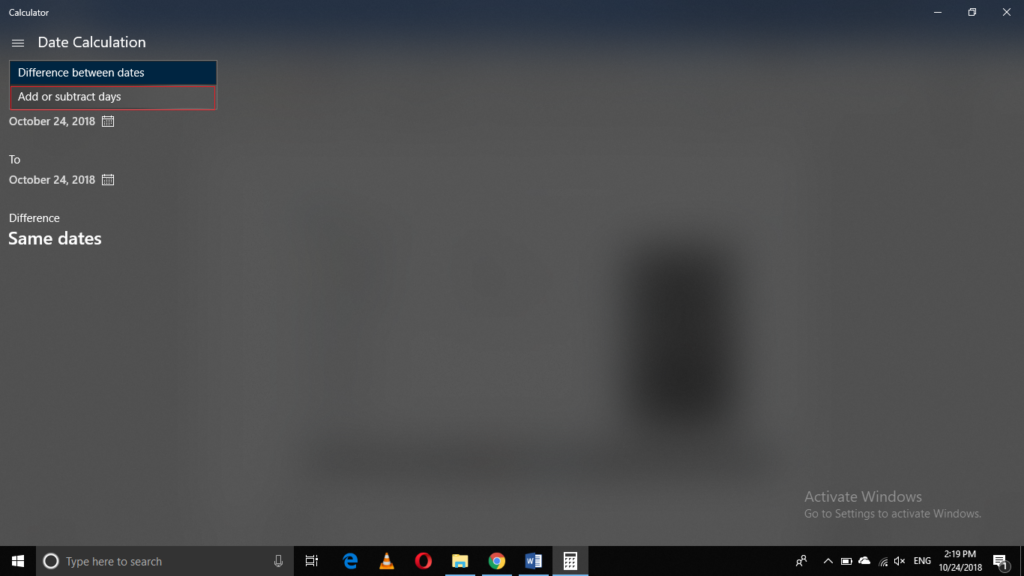As soon as you will click on it, the interface of the Calculator app will change as shown in the image below:Now you can choose any of the radio buttons corresponding to the Add and Subtract fields in order to perform addition or subtraction operations respectively. You can choose any date of your choice from the calendar associated with the “From” field to which you want to add or subtract days. Finally, you can choose the number of days you want to add or subtract by using the dropdown lists corresponding to the fields of Years, Months and Days respectively.

In this example, I am going to add 60 days to the current date as highlighted in the following image: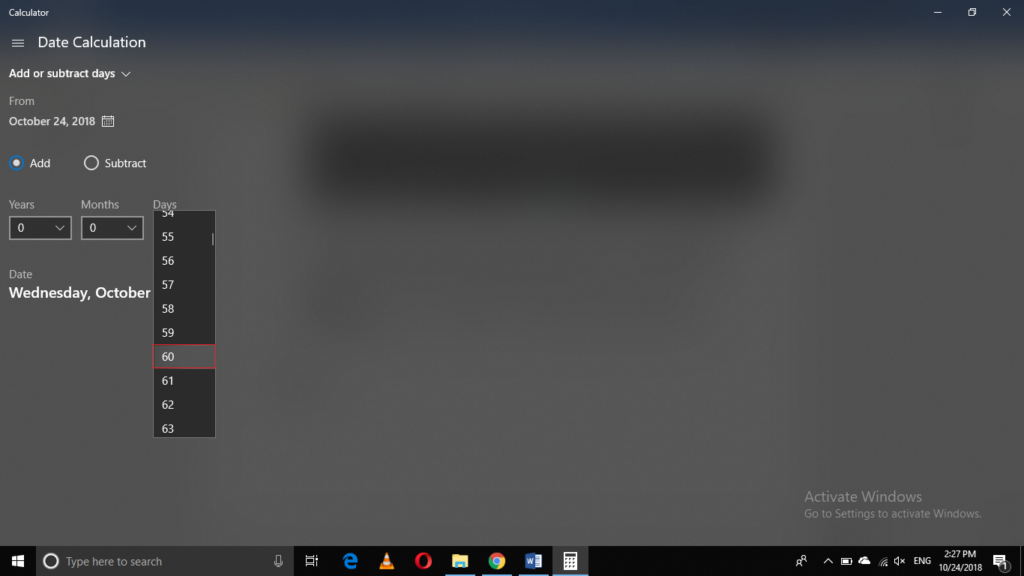As soon as you will select the number of days, the new date will appear on the screen which is obtained by adding 60 days to the current date as highlighted in the image shown below: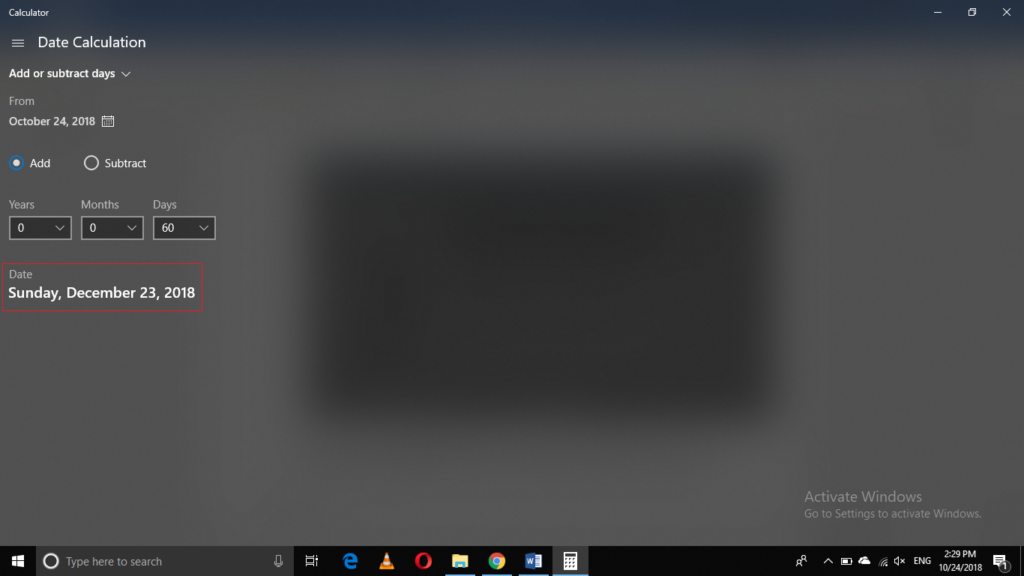## Conclusion

In this way, you can perform tedious date calculations within nanoseconds and that too without any difficulty using the Calculator app in Windows 10. This app is so convenient and easy to use that it hardly takes a few minutes to learn it and then you can do wonders with it.